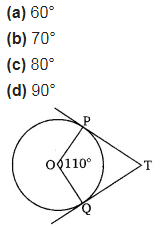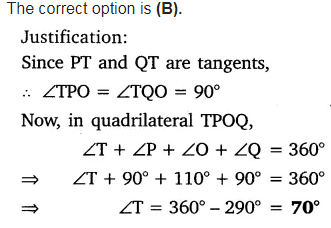# In the figure, if TP and TQ are the two tangents to a circle with center O

In the figure, if TP and TQ are the two tangents to a circle with center O so that ∠POQ = 110°, then ∠PTQ is equal to